Courses

# RD Sharma Solutions -Ex-7.6, Decimals, Class 6, Maths Class 6 Notes | EduRev

## RD Sharma Solutions for Class 6 Mathematics

Created by: Abhishek Kapoor

## Class 6 : RD Sharma Solutions -Ex-7.6, Decimals, Class 6, Maths Class 6 Notes | EduRev

The document RD Sharma Solutions -Ex-7.6, Decimals, Class 6, Maths Class 6 Notes | EduRev is a part of the Class 6 Course RD Sharma Solutions for Class 6 Mathematics.
All you need of Class 6 at this link: Class 6

Question 1

Express as rupees (Rs) using decimals

i) 15 paisa

We know that 100 paisa = Rs. 1

Therefore, 1 paisa = Rs.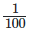15 paisa =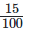= Rs 0.15

ii) 5 paisa

We know that 100 paisa = Rs. 1

Therefore, 1 paisa = Rs.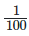5 paisa =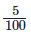= Rs 0.05

iii) 350

We know that 100 paisa = Rs. 1

Therefore, 1 paisa = Rs.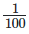35. paisa =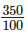= Rs 3.50

iv) 2 rupees 60 paisa

We know that 100 paisa = Rs. 1

Therefore, 1 paisa = Rs.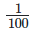2 rupees 60 paisa = 2+60100

= Rs 2.60

Question 2

Express as metres (m) using decimals

i) 15 cm

We know that 100 cm = 1m

Therefore 1 cm =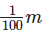15cm = 15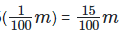= 0.15 m

ii) 8 cm

We know that 100 cm = 1m

Therefore 1 cm =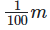8cm = 8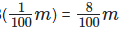= 0.08 m

iii) 135 cm

We know that 100 cm = 1m

Therefore 1 cm =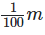135cm = 135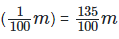= 1.35m

iv) 3m 65 cm

We know that 100 cm = 1m

Therefore 1 cm =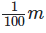3m 65cm = 3+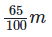= 3.65 m

Question 3

Express as centimetre (cm) using decimals

i) 5 mm

We know that 10 mm = 1cm

Therefore 1 mm =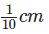5 mm =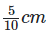= 0.5 cm

ii) 60mm

We know that 10 mm = 1cm

Therefore 1 mm =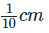60 mm =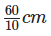= 6 cm

iii) 175 mm

We know that 10 mm = 1cm

Therefore 1 mm =175 mm =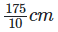= 17.5 cm

iv) 4 cm 5 mm

We know that 10 mm = 1cm

Therefore 1 mm =4cm 5 mm = 4+510

= 4.5 cm

Question 4

Express as kilogram (km) using decimals

i) 5 m

We know that 1000m = 1 km

Therefore 1m =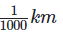5m =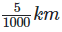= 0.005 km

ii) 55m

We know that 1000m = 1 km

Therefore 1m =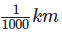55m =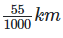= 0.055 km

iii) 555m

We know that 1000m = 1 km

Therefore 1m =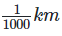555m =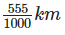= 0.555 km

iv) 5555m

We know that 1000m = 1 km

Therefore 1m =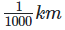5m =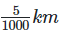= 5.555 km

v) 15 km 35 m

We know that 1000m = 1 km

Therefore 1m =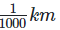15km35m = 15+351000

= 15.035 km

Question 5

Express each of the following without using decimals

i) 8g

We know that 1000g = 1kg

Therefore 1 g =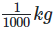= 0.001 kg

8g =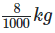= 0.008 kg

ii) 150 g

We know that 1000g = 1kg

Therefore 1 g =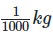= 0.001 kg

150g =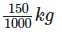= 0.150 kg

iii) 2750 g

We know that 1000g = 1kg

Therefore 1 g == 0.001 kg

2750g =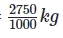= 2.750 kg

iv) 5 kg 750 g

We know that 1000g = 1kg

Therefore 1 g =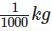= 0.001 kg

5 kg 750g = 5+7501000

= 5.750 kg

v) 36 kg 50 g

We know that 1000g = 1kg

Therefore 1 g == 0.001 kg

36kg 50g = 36+501000

= 36.050 kg

Question 6

Express each of the following without using decimals

i) Rs.5.25

We know 100 paisa = 1 rupee

So , 1 paisa =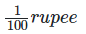Therefore, Rs 5.25 = 5+0.25

= 5+25100 = Rs5 and 25 paisa

ii) 8.354 kg

We know that 100g = 1kg

So 1 g =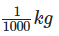Therefore, 8.354 = 8+0.354 = 8+3541000 = 8 kg 354 g

1. 3.5 cm

We know that 10 mm = 1cm

So, 1 mm =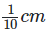Therefore 3.5 = 3+0.5

= 3+510 = 3cm 5 mm

iii) 3.05 km

We know that 1000 m = 1km

Therefore 3.05 = 3+0.05

= 3+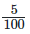= 3+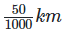= 3km 50 m

iv) 7.54 m

We know that 100 cm = 1m

Therefore 7.54= 7+0.54

= 7+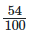= 7m 54 cm

v) 15.005 kg

We know that 1 kg =1000g

Therefore , 15.005 = 15+0.005

= 15+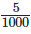= 15kg 5 g

vi) 12.05 m

We know that 1m = 100 cm

Therefore 12.05 = 12+0.05

= 12+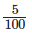= 12 m 5 cm

Offer running on EduRev: Apply code STAYHOME200 to get INR 200 off on our premium plan EduRev Infinity!

103 docs

,

,

,

,

,

,

,

,

,

,

,

,

,

,

,

,

,

,

,

,

,

,

,

,

,

,

,

,

,

,

;